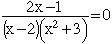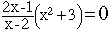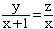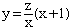Name: Susan Who is asking: Student Level: Secondary Question: I'm having a little trouble solving these equations for x. I can't seem to separate x fully from the other numbers. Please help! Here are the problems: (2x-1)/(x-2)(x 2+3) = 0 and y/(x+1)=z/x Hi Susan, If the first problem isYou can multiply bothe sides by the denominator to get 2x - 1 = 0 or x = 1/2 When you multiply both sides of an equation by some quantity you do need to ensure that the quantity you multiply by is not zero. The denominator, (x-2)(x 2+3) is zero only is x = 2 and we found that x = 1/2, hence we did not multiply by zero. If the first problem isthen multiply both sides by (x-2) to get (2x - 1)(x 2 + 3) = 0 and solve for x. For the second problemmultiply both sides by x+1 to getNext multiply both sides by x. At this point you can simplify and isolate x from the other variables. Penny Go to Math Central